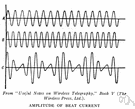# amplitude

(redirected from Amplitude (wave motion))
Also found in: Thesaurus, Medical, Legal, Encyclopedia.
Related to Amplitude (wave motion): amplitude modulation

## am·pli·tude

(ăm′plĭ-to͞od′, -tyo͞od′)
n.
1. Greatness of size; magnitude.
2. Fullness; copiousness.
3. Breadth or range, as of intelligence.
4. Astronomy The angular distance along the horizon from true east or west to the intersection of the vertical circle of a celestial body with the horizon.
5. Physics The maximum absolute value of a periodically varying quantity.
6. Mathematics
a. The maximum absolute value of a periodic curve measured along its vertical axis.
b. The angle made with the positive horizontal axis by the vector representation of a complex number.
7. Electronics The maximum absolute value reached by a voltage or current waveform.

[Latin amplitūdō, from amplus, large.]

## amplitude

(ˈæmplɪˌtjuːd)
n
1. greatness of extent; magnitude
2. abundance or copiousness
3. breadth or scope, as of the mind
4. (Astronomy) astronomy the angular distance along the horizon measured from true east or west to the point of intersection of the vertical circle passing through a celestial body
5. (Mathematics) maths Also called: argument (of a complex number) the angle that the vector representing the complex number makes with the positive real axis. If the point (x, y) has polar coordinates (r, θ), the amplitude of x + iy is θ, that is, arctan y/x. Compare modulus2 See also Argand diagram
6. (General Physics) physics the maximum variation from the zero or mean value of a periodically varying quantity
[C16: from Latin amplitūdō breadth, from amplus spacious]

## am•pli•tude

(ˈæm plɪˌtud, -ˌtyud)

n.
1. the state or quality of being ample, esp. as to breadth or width; largeness.
2. large or full measure; abundance.
3. mental range, scope, or capacity.
4. the absolute value of the maximum displacement from a zero value during one period of an oscillation.
5. the maximum deviation of an alternating current from its average value.
6. the arc of the horizon measured from the east or west point to the point where a vertical circle through a heavenly body would intersect the horizon.
[1540–50; < Latin amplitūdō. See ample, -i-, -tude]

## am·pli·tude

(ăm′plĭ-to͞od′)
One half the full extent of a vibration, oscillation, or wave. The amplitude of an ocean wave, for example, is the maximum height of the wave crest above the level of calm water, or the maximum depth of the wave trough below the level of calm water. The amplitude of a pendulum swinging through an angle of 90° is 45°. See more at wave.

## amplitude

A wave’s greatest displacement from equilibrium.
ThesaurusAntonymsRelated WordsSynonymsLegend:
 Noun 1amplitude - (physics) the maximum displacement of a periodic wavenatural philosophy, physics - the science of matter and energy and their interactions; "his favorite subject was physics"shift, displacement - an event in which something is displaced without rotation 2amplitude - the property of copious abundance  abundance, copiousness, teemingness - the property of a more than adequate quantity or supply; "an age of abundance" 3 amplitude - greatness of magnitudemagnitude - the property of relative size or extent (whether large or small); "they tried to predict the magnitude of the explosion"; "about the magnitude of a small pea"signal level - the amplitude level of the desired signalbackground level, noise level - the amplitude level of the undesired background noise

## amplitude

noun
1. a man of great amplitude
2. The character comes to imply an amplitude of meanings.

## amplitude

noun
1. Great extent, amount, or dimension:
bulk, magnitude, mass, size, volume (often used in plural).
2. The quality or state of being large in amount, extent, or importance:
Translations
amplituda
amplitudilaajuus
amplituda
amplitude
amplitudmagnitud

[ˈæmplɪtjuːd] N

## amplitude

[ˈæmplɪtjuːd] n
(= strength) [soundwave, signal] →
(= largeness) →

## amplitude

n (of knowledge)Weite f, → Breite f; (of bosom)Üppigkeit f, → Fülle f; (Phys) → Amplitude f

## amplitude

[ˈæmplɪˌtjuːd] n (Math, Phys) → ampiezza
Site: Follow: Share:
Open / Close